[TOC]

# 1. API and elementary implementations

Collection: data struct for inserting and deleting items (ex. stack and queue).
Priority queue: a special kind of collection — remove largest/smallest element.

API:

```public class Max<Kye implements Comparable<Key>>{
public MaxPQ();
public void insert(Key k);
public Key delMax();
public boolean isEmpty();
public Key max();
int size();

}
```

## PQ client example

find M largest elements from N items. (N is too huge to store)
⇒ 思路: 用 MinPQ, 当size>M时删掉最小元素 — 最后剩下的就是最大的M个元素了.## elementary(naive) implementations

unordered array implementation
insert ~1,
remove ~N

ordered array implementation
insert ~N — ~ insertion sort,
remove ~1

goal:
insert O(lgN) & remove max O(lgN)

# 2. Binary Heaps

binary heap: a special kind of complete binary tree.

def. "complete binary tree"
All level except the last level are full, all nodes in the last level are as far left as possible.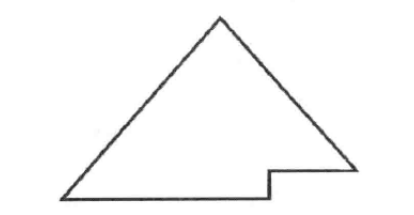property: a complete binary tree with N items has height = lgN.

def. binary heap
Binary heap is a complete binary tree that satisfies: each node >= any of its 2 children ("heap ordering").

property of binary heap:

• max element is root
• height is lgN
• each node still makes a heap (use this property to construct heap from bottom up)

## Array representation of binary heap

Use an array `a[]` to represent a complete binary tree: very easy to get children and parent.

• index starting from 1 (root = a, do not use a)
• for node at index `i`, its children are: `i*2` and `i*2+1`
• for node at index `i` (i>1), its parent is: `i/2`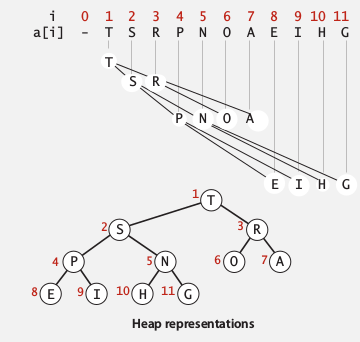## Implementation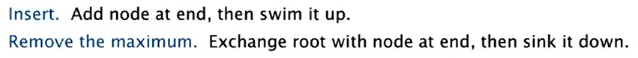```public class MaxPQ<Key implements Comparable<Key>>{
private Key[] a;
private int N;
}
```

siftup (swim)
if a node is larger than its parent: exchange with parent until heap ordering is restored.

```private void swim(int k){
while(k>1 && less(a[k/2], a[k]){
exch(a,k,k/2);
k /= 2;
}
}
```

use siftup for inserting elements
when inserting an element, first append it to the end of the array, then siftup the element to its right position.

```public void insert(Key k){
a[N++]=x;
swim(N);
}
```

siftdown (sink)
if a node is smaller than its children: exchange it with the bigger child. 用公司高层变动来类比很形象...

```private void sink(int k){
while(k*2<=N){
int maxIndex = less(a[k],a[k*2])? k*2 : k;
if(k*2+1<=N && less(a[maxIndex],a[k*2+1]) maxIndex = 2*k+1;
if(maxIndex==k) break;
exch(a,k,maxIndex);
k = maxIndex;
}
}
```

⇒ use siftdown for deleting max operation
First put a[N] to the position of root, then sink. (注意要防止loitering)

```public Key delMax(){
Key ret = a;
a = a[N];
a[N--] = null; // avoid loitering
sink(1);
return ret;
}
```

## Analysis

insert: ~lgN
remove max: ~lgN

improvements:

• use a d-way heap instead of a 2-way heap (for ex. 3-way heap, the children of i are i3, i3+1, i*3+2, the parent of i is i/3)
• Fibonacci heap: insert is ~1, remove max still ~lgN, (but too complicated to use in practice).considerations:

• use immutable keys to avoid client from changing the keys (`final` keyword in java)
• underflow/overflow: throw exceptions for delMax() when empty, use resizing array for implementation.
• expand the API with other operations: remove an arbitrary item, change the prority of an item (like handling an array)...

# 3. Heapsort

Basic idea:

• create a max heap with all N entries ("heap-construction")
• then repeatedly remove all N items ("sortdown")

## Implementation

heap construction
Construct the heap using a bottom-up method: build the heaps with small size first.

• one-node heaps (jus leaf node of heap): need not consider
• larger heaps: children(subtrees) are already heap-order → just perform sink operation for root

sink elements at indices from N/2 to 1

```for(int k=N/2; k>=1; k++)
sink(k);
```

→ complexity is linear, see analysis below.

heap destruction
now that we have a max-heap, to get the sorted array in-place, simply do:

```while(N>1){
exch(a,1, N--);
sink(1);
}
```

## Analysis

proposition
Heap construction uses <2N compares and exchanges.
proof

→ 每一层最多有 k*2^(h-k) 次交换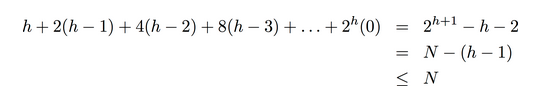(第一个等式可以用数学归纳法证...)

proposition
Heapsort use < 2NlgN compares and exchanges.

Heapsort is the first in-place sorting algorithm with NlgN worst-case performance.⇒ heapsort is optimal for time and space, but:

• inner loop is longer than quicksort
• poor use of cache memory (too many references to cache — look far away from the array entry when going down through the tree)
• not stable: because it does long-distance exchanges

Summary: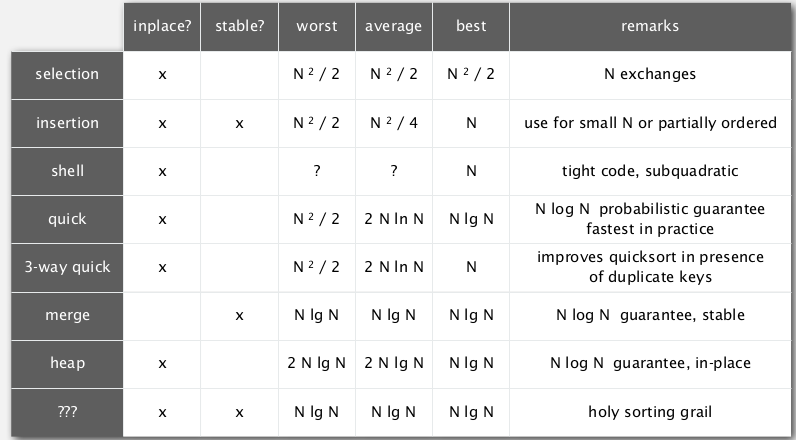# 4. Event-driven simulation

Goal: simulating the motion of N particles in elastic collision (using a priority queue).## model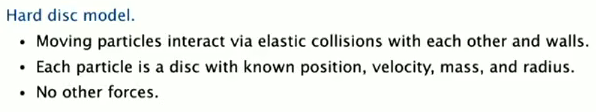bouncing balls (without collision)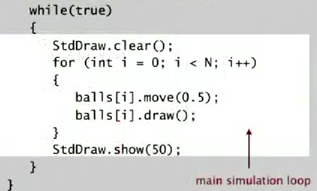Ball class: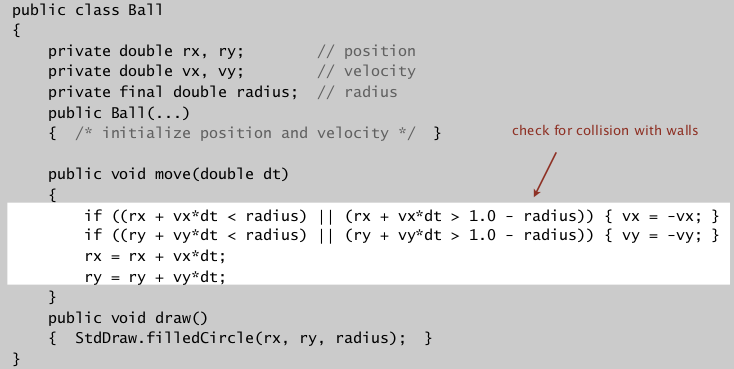challenge: which objects to check? How to do it efficently ?

Time driven simulation

• upate the position of balls every `dt` seconds
• if overlap detected: figure how the velocity change and continue the simulation.

problem with time-driven simulation:

• 1/2*N^2 overlap check per time unit
• if dt small: too much calculation
• if dt too large: might miss collisions

## Event driven simulation

change state only when something happens

• particles move in straight line before collision
• → collision time can be predicted
• maintain PQ for collisions, key=collision time

collision prediction
input: each particle has radius `s`, position `(rx, ry)`, velocity `(vx, vy)`.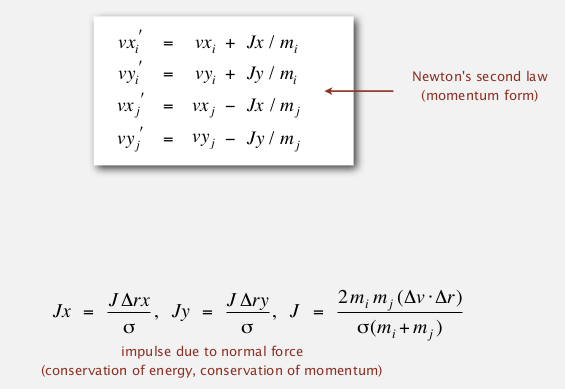(美国高中物理这么凶残?...)

## Implementation

anyway, 这个是封装好的类: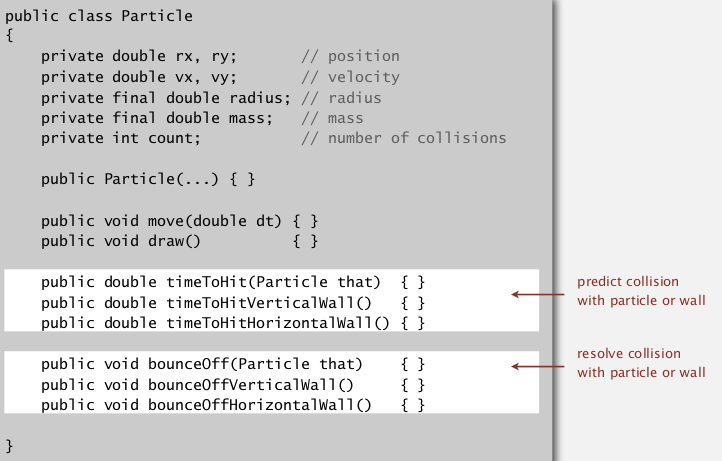initialization: quadratic

• Fill PQ with all potential particle-particle collisions
• Fill (the same) PQ with all potential particle-wall collisions

Main loop

• get next event from PQ, event time = t
• ignore if the event is invalidated
• advance all particles to time t
• update velocities
• predict future collisions and insert to PQ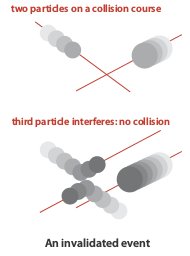(详见他们的代码: http://algs4.cs.princeton.edu/61event/CollisionSystem.java.html)

Event class: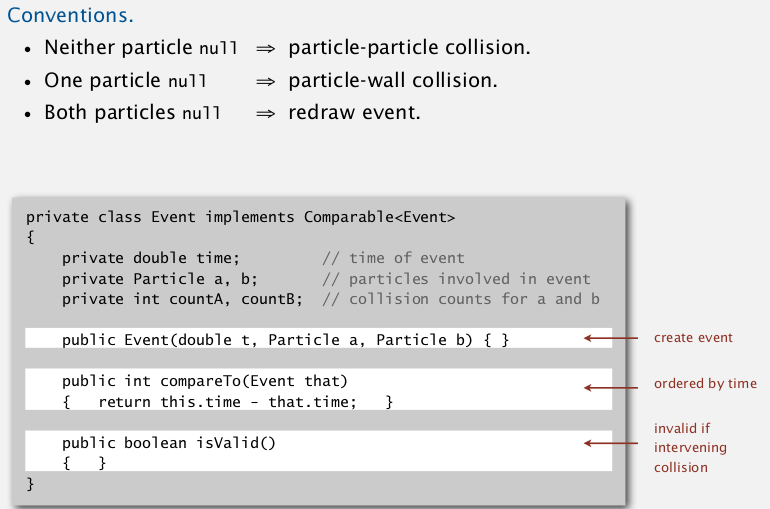#### Part 8 of series «Algorithms Princeton MOOC I»：

comments powered by Disqus